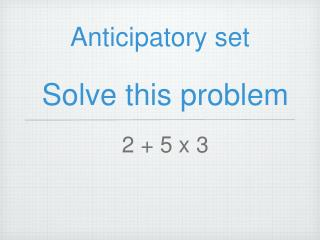DownloadDownload PresentationSolve this problem

# Solve this problem

Download Presentation## Solve this problem

- - - - - - - - - - - - - - - - - - - - - - - - - - - E N D - - - - - - - - - - - - - - - - - - - - - - - - - - -
##### Presentation Transcript

1. Anticipatory set Solve this problem • 2 + 5 x 3

2. Are there two right answers? • There is only one right answer because of the correct order of operations. • The right answer is 17.

3. Order of Operation • I can use order of operations to simplify numerical expressions.

4. Essential Questions • What is the correct order of operations? • Why is it important to apply the correct order of operations when solving numerical expressions?

5. PEMDASD • P Do all parentheses first • E Apply any exponents • MD Do all multiplication and division, working from left to right • AS Do all addition and subtraction, working from left to right • D or F Division Bar or Fraction Bar (Simplify Fractions)

6. Be Careful • be careful to work Multiplication and division from left to right. • The right way The wrong way • 12 ÷ 3 x 2 12 ÷ 3 x 2 • 4 x 2 12 ÷ 6 • 8 2

7. Watch me! • 5 + 4 + 7 x 3 • 5 + 4 + 21 • 9 + 21 30

8. Watch me! • 28 ÷ 4 - (4 + 3) • 28 ÷ 4 – 7 • 7 - 7 0

9. Let’s do some together • 22 + 16 - 8 • 4 + 16 - 8 • 20 - 8 • 12

10. Let’s do some together • (6 + 4)2 x 8 • ( 10 )2 x 8 • 100 x 8 • 800

11. Try it • 6 + 7 x 8 = • 16 ÷ 8 - 2 = • (25 - 11) x 3 = • 9 x 4 - 6 ÷ 3 = • 16 ÷ 4 x 2 = • 2 x 9 ÷ 3 = • 9 + 6 x (8 - 5) = Bonus: 2 x (5 + 4) ÷ 32 - 1

12. Closure • Close your notes • Think about the correct order or operations • Write the correct order on your slate

13. Order of Operation • I can use order of operations to simplify numerical expressions.

14. Essential Questions • What is the correct order of operations? • Why is it important to apply the correct order of operations when solving numerical expressions?

15. PEMDAS • P Do all parentheses first • E Apply any exponents • MD Do all multiplication and division, working from left to right • AS Do all addition and subtraction, working from left to right • D Division Bar or Fraction Bar (Simplify Fractions)

16. Be Careful Be careful to work Multiplication and division from left to right. The right way The wrong way

17. Watch me! 5 + 4 + 7 x 3

18. Watch me! • 28 ÷ 4 - (4 + 3)

19. Let’s do some together • 22 + 16 - 8

20. Let’s do some together (6 + 4)2 x 8

21. Try it • 6 + 7 x 8 = • 16 ÷ 8 - 2 = • (25 - 11) x 3 = • 9 x 4 - 6 ÷ 3 = • 16 ÷ 4 x 2 = • 2 x 9 ÷ 3 = • 9 + 6 x (8 - 5) = Bonus: 2 x (5 + 4) ÷ 32 - 1